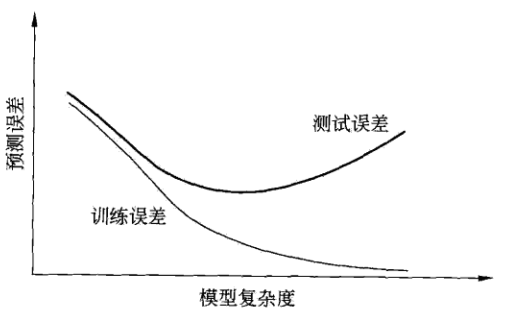# 统计学习的三要素

1. 模型
2. 策略
3. 算法

## 策略

### 损失函数和风险函数

1. 0-1损失函数：
$L(Y,f(X))= 1, Y \ne f(X)$ ; $L(Y,f(X))= 0, Y = f(X)$
2. 平方损失函数:
$L(Y,f(X)) = (Y-f(X))^2$
3. 绝对损失函数：
$L(Y,f(X)) = |Y-f(X)|$
4. 对数损失函数：
$L(Y,f(X)) = - \lg P(Y|X)$

$R(f) = E_p [L(Y,f(X))]= \int L(y,f(x)) P(x,y) dxdy$

$R(f) = \frac{1}{N} \sum L(y_i,f(x_i))$

### 经验风险最小化与结构风险最小化

$\min_{f \in F} \frac{1}{N} \sum L(y_i,f(x_i))$

$R(f) = \frac{1}{N} \sum L(y_i,f(x_i)) + \lambda J(f)$

# 模型评估与模型选择

## 训练误差和测试误差

$R_{emp} (\hat{f}) = \frac{1}{N} \sum_i ^{N} L(y_i,\hat{f}(x_i))$

$e_{test} = \frac{1}{N’} \sum_i ^{N’} L(y_i,\hat{f}(x_i))$

## 过拟合与模型选择# 生成模型与判别模型

1. 生成方法可以还原出联合概率分布$P(X,Y)$， 而判别方法则不能；
2. 生成方法的学习收敛速度更快，即当样本容量增加的时候，学到的模型可以更快地收敛于真实的模型；
3. 当存在隐变量的时候，仍可以用生成方法学习，此时判别方法就不能用。

1. 判别方法学习的是条件概率$P(Y|X)$或决策函数$f(X)$,直接面对预测，往往学习的准确率更高；
2. 由于直接学习$P(Y|X)$或$f(X)$，可以对数据进行各种程度上的抽象、定义特征并使用特征，因此可以简化学习问题。

# 参考文献

1. 李航.统计学习方法.清华大学出版社.2012
2. Peter Harrington.机器学习实战.人民邮电出版社.2013